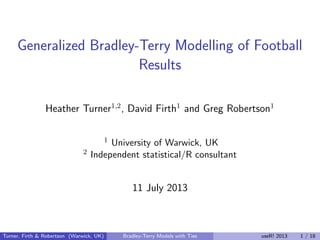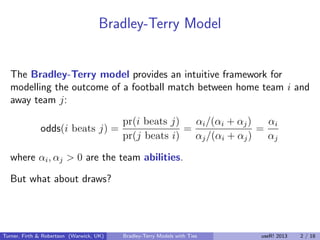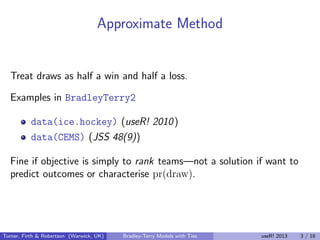Successfully reported this slideshow.

# Generalized Bradley-Terry Modelling of Football Results×

# Generalized Bradley-Terry Modelling of Football Results

Generalization of the Davidson model for modelling paired comparisons, to incorporate a tied outcome, applied to the results of football (soccer) results from the English first division.

Generalization of the Davidson model for modelling paired comparisons, to incorporate a tied outcome, applied to the results of football (soccer) results from the English first division.

### Generalized Bradley-Terry Modelling of Football Results

1. 1. Generalized Bradley-Terry Modelling of Football Results Heather Turner1,2 , David Firth1 and Greg Robertson1 1 University of Warwick, UK 2 Independent statistical/R consultant 11 July 2013 Turner, Firth & Robertson (Warwick, UK) Bradley-Terry Models with Ties useR! 2013 1 / 18
2. 2. Bradley-Terry Model The Bradley-Terry model provides an intuitive framework for modelling the outcome of a football match between home team i and away team j: odds(i beats j) = pr(i beats j) pr(j beats i) = αi/(αi + αj) αj/(αi + αj) = αi αj where αi, αj > 0 are the team abilities. But what about draws? Turner, Firth & Robertson (Warwick, UK) Bradley-Terry Models with Ties useR! 2013 2 / 18
3. 3. Approximate Method Treat draws as half a win and half a loss. Examples in BradleyTerry2 data(ice.hockey) (useR! 2010) data(CEMS) (JSS 48(9)) Fine if objective is simply to rank teams—not a solution if want to predict outcomes or characterise pr(draw). Turner, Firth & Robertson (Warwick, UK) Bradley-Terry Models with Ties useR! 2013 3 / 18
4. 4. Davidson Model The Davidson model extends the Bradley-Terry model to incorporate the probability of a draw: pr(draw) = ν √ αiαj αi + αj + ν √ αiαj pr(i beats j | not a draw) = αi αi + αj where ν > 0. Like the Bradley-Terry model, this can be expressed as a log-linear model and estimated using glm. Turner, Firth & Robertson (Warwick, UK) Bradley-Terry Models with Ties useR! 2013 4 / 18
5. 5. Closer Look at ν ν → ∞: pr(draw) → 1 ν → 0: pr(draw) ∝ ν √ αiαj/(αi + αj) (approx.) The single extra parameter ν conﬂates overall (max) probability of a draw strength of dependence of pr(draw) on αi, αj. Turner, Firth & Robertson (Warwick, UK) Bradley-Terry Models with Ties useR! 2013 5 / 18
6. 6. Dependence of pr(draw) on Relative Ability Under the Davidson model we have logit (pr(draw)) = log ν √ αiαj αi + αj = log ν αi αi + αj 1 2 αj αi + αj 1 2 = log νp 1 2 (1 − p) 1 2 where p = pr(i beats j | not a draw) This enables us to visualise the dependence of pr(draw) on the relative ability of the teams. Turner, Firth & Robertson (Warwick, UK) Bradley-Terry Models with Ties useR! 2013 6 / 18
7. 7. 0.0 0.2 0.4 0.6 0.8 1.0 0.00.20.40.60.81.0 Davidson (1970) model for ties, for 1/4 < nu < 128 pr(i beats j in a non−tied contest) pr(iandjtie) Turner, Firth & Robertson (Warwick, UK) Bradley-Terry Models with Ties useR! 2013 7 / 18
8. 8. Home Advantage So far based model purely on team ability. In football (as other sports) expect home advantage to boost ability of home team, i: pr(draw) = ν √ µαiαj µαi + αj + ν √ µαiαj pr(i beats j | not a draw) = µαi µαi + αj Turner, Firth & Robertson (Warwick, UK) Bradley-Terry Models with Ties useR! 2013 8 / 18
9. 9. Generalised Davidson Model We propose the following generalisation of the Davidson model: logit (pr(draw)) = log δ c pσπ (1 − p)σ(1−π) where c is function of σ, π such that expit(δ) is the maximum probability of a draw σ scales the dependence on relative ability 0 < π < 1 is the value of p with maximum pr (draw)—if π = 0.5, implies home advantage eﬀect. Turner, Firth & Robertson (Warwick, UK) Bradley-Terry Models with Ties useR! 2013 9 / 18
10. 10. Changing σ 0.0 0.2 0.4 0.6 0.8 1.0 0.00.20.40.60.81.0 σ = 0.1 pr(i beats j | not a draw) pr(draw) 0.0 0.2 0.4 0.6 0.8 1.0 0.00.20.40.60.81.0 σ = 1 pr(i beats j | not a draw) pr(draw) 0.0 0.2 0.4 0.6 0.8 1.0 0.00.20.40.60.81.0 σ = 10 pr(i beats j | not a draw) pr(draw) Turner, Firth & Robertson (Warwick, UK) Bradley-Terry Models with Ties useR! 2013 10 / 18
11. 11. Changing π 0.0 0.2 0.4 0.6 0.8 1.0 0.00.20.40.60.81.0 π = 0.25 pr(i beats j | not a draw) pr(draw) 0.0 0.2 0.4 0.6 0.8 1.0 0.00.20.40.60.81.0 π = 0.5 pr(i beats j | not a draw) pr(draw) 0.0 0.2 0.4 0.6 0.8 1.0 0.00.20.40.60.81.0 π = 0.75 pr(i beats j | not a draw) pr(draw) Turner, Firth & Robertson (Warwick, UK) Bradley-Terry Models with Ties useR! 2013 11 / 18
12. 12. English Premier League Results Consider data from the past 5 seasons of the English Premier League 20 teams, 380 games/season ≈ 1 4 games drawn First convert the the win/lose/draw results to trinomial counts football.tri <- expandCategorical(football, "result", idvar = "match") head(football.tri) home away season result match count 1 Ars Ast 2008-9 -1 1 1 2 Ars Ast 2008-9 0 1 0 3 Ars Ast 2008-9 1 1 0 4 Ars Ast 2010-11 -1 2 1 5 Ars Ast 2010-11 0 2 0 6 Ars Ast 2010-11 1 2 0 Turner, Firth & Robertson (Warwick, UK) Bradley-Terry Models with Ties useR! 2013 12 / 18
13. 13. Generalised Davidson as Poisson Model Model log expected counts for each match k (log probabilities) log(pr(i beats j)k) = θijk + log(µαi) log(pr(draw)k) = θijk + log δ − log c + σ(π log(µαi) − (1 − π) log(αj)) + (1 − σ) log(µαi + αj) log(pr(j beats i)k) = θijk + log(αj) where θijk ﬁxes the total count for each match to 1. With gnm: gnm(count ~ GenDavidson(result == 1, result == 0, result == -1, home:season, away:season, home.adv = ~1, tie.max = ~1, tie.scale = ~1, tie.mode = ~1, at.home1 = home1, at.home2 = home2) - 1, eliminate = match, family = poisson, data = football.tri) Turner, Firth & Robertson (Warwick, UK) Bradley-Terry Models with Ties useR! 2013 13 / 18
14. 14. Alternative Models We consider the following models 1 Davidson: σ = 1, π = 0.5 2 Scaled Davidson: σ = ˆσ, π = 0.5 3 Shifted, scaled Davidson: σ = ˆσ, π = ˆπ Resid. Df Resid. Dev Df Deviance Pr(>Chi) 1 3665 3533.7 2 3664 3523.2 1 10.4657 0.001216 ** 3 3663 3517.6 1 5.5575 0.018402 * Turner, Firth & Robertson (Warwick, UK) Bradley-Terry Models with Ties useR! 2013 14 / 18
15. 15. q qqq q q q q q q q q q q q q q q q qq q q q q q q q q q q q q q q q q q q q q q q q q q q q q qqqq q q qq q q q q q qq qqq qq Davidson Proportion q q Non−drawn matches won Matches drawn q qq qq qq qq q q q qq q q q q q q q q q q q q qqq q q q q q q q q q q q q q q q q q q q q q q q q q q q q q q q q q q qq q qq q q q q q q q q q q q q q q q q q q q q qq q q q q pr(i beats j | not a draw) 0.0 0.5 1.0 0.000.321.00 σ = 1, q = 0.5 Turner, Firth & Robertson (Warwick, UK) Bradley-Terry Models with Ties useR! 2013 15 / 18
16. 16. q q q q qq q q q q q q q q q q q q q q q q q q q q q q q q qq qqq q q q q q q q q q q q qqq q q q q q q q q q q qqq q qq q qq q Scaled Davidson Proportion q q Non−drawn matches won Matches drawn q q q qq qq q q q q qq q q q q q q q q q qq q q q q q q q q q qq q q q q q q qqq q q q q q q q q q qq q q q q q q q qq q qq q q q q q q qqqq q qq q q q q q qq q q q qq q q pr(i beats j | not a draw) 0.0 0.5 1.0 0.000.351.00 σ = 2.22, q = 0.5 Turner, Firth & Robertson (Warwick, UK) Bradley-Terry Models with Ties useR! 2013 16 / 18
17. 17. q q q q q q q q q q q q q q q q q q q qq q q q q qq q q q q q q q q q q q q q q q q q qq q q q q q q q q q qq q q q q q q qq q qq q Shifted & Scaled Davidson Proportion q q Non−drawn matches won Matches drawn q q q q qqq q q qq q q q q q q qq q q qq qq q q qq q qqq qq q q q q q qq q q q q q qq q q q q q q q q q q q q q q q q qq q q q q q q q qq q q q q q q q q q qq qq q qq q q pr(i beats j | not a draw) 0.00 0.58 1.00 0.000.361.00 σ = 3.12, q = 0.58 Turner, Firth & Robertson (Warwick, UK) Bradley-Terry Models with Ties useR! 2013 17 / 18
18. 18. Conclusions Proposed model explicitly models draws distinguishes overall pr(draw) from dependence of pr(draw) on relative ability Expressing pr(draw) in terms of pr(i beats j | not a draw) helps to assess model ﬁt. plotProportions and GenDavidson to be provided as example in BradleyTerry2. Turner, Firth & Robertson (Warwick, UK) Bradley-Terry Models with Ties useR! 2013 18 / 18